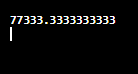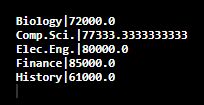# Aggregate functions in SQL

## Aggregate Functions:

Aggregate functions take a set of values as input and return a single value. SQL offers five aggregate functions:

1. Average: avg
2. Minimum: min
3. Maximum: max
4. Total: sum
5. Count: count

The input to sum and avg function is a collection of numbers, but the other operators can be a collection of non-numeric data types, such as strings, as well.

Consider table instructors to perform aggregate functions in SQL:

 ID name dept_ name salary 76766 Crick Biology 72000 45565 Katz Comp. Sci. 75000 10101 Srinivasan Comp. Sci. 65000 83821 Brandt Comp. Sci. 92000 98345 Kim Elec. Eng. 80000 12121 Wu Finance 90000 76543 Singh Finance 80000 32343 El-Said History 60000 58583 Califieri History 62000

### Average – avg function:

This function performs average for a given database.

For example, to know the average salary in the Computer Science department. The corresponding SQL query will be:

select avg (salary)
from instructors
where dept_name= ’Comp. Sci.’;

### Output:### Minimum – min function:

This function finds a minimum of a given database. For example, for the above database ‘instructors’ we write the minimum SQL query as follows:

select min(salary) from instructors
where dept_name = 'Comp.Sci.';

### Output:### Maximum – max function:

It is used to find the maximum of a given database.

For example, for the above database ‘instructors’ we write the maximum SQL query as follows:

select max(salary) from instructors
where dept_name = 'Comp.Sci.';

### Output:### SUM- sum function:

This is used to find a total of a given database.

The query for SUM aggregate function in SQL is:

select sum(salary) from instructors
where dept_name = 'Comp.Sci.';

### Output:### COUNT- count function:

We use count, the aggregate function to count the number of tuples in a table. It is denoted in SQL as count (*).

select count (*)
from instructors;

### Output:## Aggregation with Grouping:

### Group by clause:

There are situations where we must apply the aggregate function to a group of sets of tuples. So we use the group by clause. Tuples with the same value are placed in one group.

For example, To know the average salary in the distinct department”. The SQL query will be:

select dept_name, avg(salary)
from instructors
group by dept_name;


### Output:### The Having Clause:

In SQL we have the having clause after groups have been formed. The having clause in SQL can be written as:

select dept_name, avg (salary)
from instructors
group by dept_name
having avg(salary) > 70000;


### Output: